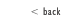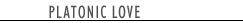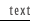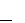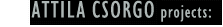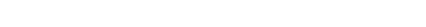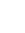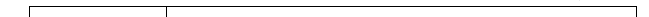Platonic Love There are only five regular solids in the universe: tetrahedron, cube, octahedron, dodecahedron and icosahedron. The first known description of these solids is found in the writings of Plato, hence the name “Platonic solids”. They all have a “neat” structure: they can all be inscribed within a sphere, and in each solid, all planes, edges and vertices are of the same size. This unique regularity may have inspired Plato to attribute a cosmological significance to these solids: he associated four of the solids with the four elements, while, in his system, the fifth became the foundation of the world. Platonic Love is based on particular aspects of geometrical correspondence between the first two of the five solids, which I discovered by performing a simple mathematical equation: I counted the number of edges in a tetrahedron and in a cube. I found that a tetrahedron has exactly half as many edges as a cube. I therefore established the equation that 1 tetrahedron + 1 tetrahedron = 1 cube, as an experimental geometrical formula. In order to create a visual representation, I constructed a moving model. The symmetrically positioned tetrahedra slowly unite into a cube, then the cube splits into two tetrahedra again. The duration of the restructuring between the two end stages is significantly longer than the moment in which the solids appear as distinct forms. This intermediate stage appears relatively unstructured, in that it displays a character which is difficult to define. If the motion were to be frozen in this intermediate stage, there would be little to suggest that the jumble of edges had any connection with a regular solid. The tetrahedron and the cube are precisely defined solids, and therefore represent timeless geometrical relationships. They are connected by the temporality of the metamorphosis. The decreasing/increasing confusion of the intermediate forms does not satisfy the topology of regularity; their irregularity is, however, the result of a precisely calculated motion. Extracted from the continuity of motion, they might be regarded as half solids, or the embryonic forms of solids. If one looks instead at the continuity of motion, the whole of the metamorphosis may be regarded as a multi-directional, pulsating body, with two timeless forms at its end stages. << top of the page